# Variance and average

Of the 40 values were calculated average mx = 7.5 and variance sx = 2.25. After the control was found to lack the two items of the values of x41 = 3.8 and x42=7. Correct the above characteristics (mx and sx).

m2 =  7.4
r2 =  2.4552

### Step-by-step explanation:Did you find an error or inaccuracy? Feel free to write us. Thank you!Tips to related online calculators
Looking for help with calculating arithmetic mean?
Looking for a statistical calculator?

#### You need to know the following knowledge to solve this word math problem:

We encourage you to watch this tutorial video on this math problem:

## Related math problems and questions:

• MeanA student's food the mean of 50 items as 38.6. When checking the work, he found that he had taken one item as 50 to read as 40 in these circumstances correctly. What should be the correct mean?
• The rawThe raw data presented here are the scores (out of 100 marks) of a market survey regarding the acceptability of new product launched by a company for random sample of 50 respondents: 40 45 41 45 45 30 30 8 48 25 26 9 23 24 26 29 8 40 41 42 39 35 18 25 35
• SD - meanThe mean is 10 and the standard deviation is 3.5. If the data set contains 40 data values, approximately how many of the data values will fall within the range of 6.5 to 13.5?
• N percentille problemHere is a data set (n=117) that has been sorted. 10.4 12.2 14.3 15.3 17.1 17.8 18 18.6 19.1 19.9 19.9 20.3 20.6 20.7 20.7 21.2 21.3 22 22.1 22.3 22.8 23 23 23.1 23.5 24.1 24.1 24.4 24.5 24.8 24.9 25.4 25.4 25.5 25.7 25.9 26 26.1 26.2 26.7 26.8 27.5 27.6 2
• Speed of Slovakian trainsRudolf decided to take the train from the station 'Ostratice' to 'Horné Ozorovce'. In the train timetables found train Os 5409 : km 0 Chynorany 15:17 5 Ostratice 15:23 15:23 8 Rybany 15:27 15:27 10 Dolné Naštice 15:31 15:31 14 Bánovce nad Bebravou 15:35 1
• Average ageThe company of five people has an average age of 46 years. The average age of the first four is 43 years. How many years has the fifth member of this company?
• MarketingYear; money spent on advertising; profit 2008 2 12 2009 5 20 2010 7 25 2011 11 26 2012 15 40 1. draw a scatter diagram depicting the data. 2. calculate the Pearson's correlation coefficient. 3. determine the linear regression equation.
• Controller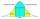Output Controller of the company in the control of 50 randomly selected products found that 37 of them had no defects, 8 has only one flaw, three had two defects, and two products had three defects. Determine the standard deviation and coefficient of vari
• First class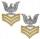The shipment contains 40 items. 36 are first grade, 4 are defective. How many ways can select 5 items, so that it is no more than one defective?
• Young mathematician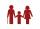One young mathematician was bored again. He found that the average age of people in the room where the seminar equals to its count. Then his 29-year-old brother entered this room. Even then, the average age of all present was the same as the count of peop
• Unemployment rate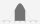Over the last 16 years, the country's unemployment rate has changed according to the following frequency table: years of unemployment: 2 5 2 3 3 1 unemployment rate: 0.5 1 1.5 2 2.5 3 in % (percent). Determine the two-sided confidence interval for the var
• Water mixingWe have 520 ml of hot water and 640 ml of water at 48°C. What is the temperature of approximately hot water when the resulting mixture has a temperature of 65°C?
• The cruise ship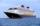The cruise ship has a speed of 12 km/h at a calm surface. When we sail 45 km along the river and 45 km back, it took us exactly 8 hours. Which (constant) speed of flow of the river?
• Box and whisker plotConstruct a box and whisker plot for the given data. 56, 32, 54, 32, 23, 67, 23, 45, 12, 32, 34, 24, 36, 47, 19, 43
• The notebook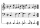After rising by 40%, the notebook cost 10.50 euros. How much did this notebook cost if it increased in price by only 20% instead of 40%?
• Average mark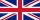Calculate average mark in English, if a student got a couple of 3's, 20% less 2 than 3, and 50% more 1's than the 2's.
• Bivariate data setHere is a bivariate data set in which you believe y to be the response variable. x y 51.4 44.8 60.2 46.6 60.1 46.8 45.8 46.3 37.4 41.8 72.4 52.5 60 48.2 67.5 49.5 60.9 49.8 51.1 45.7 62.7 49.6 194.9 84.2 Make a scatter plot of this data. Which point is an